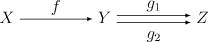# Epi and mono

My first math classes used the terms one to one and onto to describe functions. These Germanic names have largely been replaced with their French equivalents injective and surjective.

Monic and epic are the category theory analogs of injective and surjective respectively. This post will define these terms and show how they play out in several contexts. In some categories these new terms correspond exactly to their traditional counterparts, but in others they do not.

## Sets and functions

A function f from a set A to a set B is injective (one-to-one) if different points on A go to different points in B. That is, f(x) = f(y) only if xy.

The function f  is surjective (onto) if everything in B is covered. That is, for every b in B, there is some a in A such that f(a) = b.

A function is bijective if it is both injective and surjective.

## Categories and morphisms

How would we generalize these definitions to category theory? This is not obvious because the rules of category theory don’t let us peek inside objects and talk about elements. Everything has to be characterized in terms of objects and morphisms.

It turns out that the categorical way to view things is to focus on when composition can be cancelled out, either on the left or on the right.

### Epimorphisms

A morphism f from an object X to an object Y is an epimorphism if for any other object Z, and any pair of morphisms g1 and g2 from Y to Z, if g1 f = g2 f then g1 = g2. Instead of saying f is an epimorphism, some authors would say f is epic or f is an epi.If a morphism f is epic, we can cancel it out on the right.

(Unfortunately it is conventional to write function composition from right to left, like splicing in a little bit of Hebrew inside English prose. In the diagram above, f is on the left, and composition proceeds from left to right. But when we write the composition of functions we write from right to left, e.g. gf. When we say we can cancel out f on the right, we mean the right of the expression gf, not on the right of a commutative diagram!)

### Monomorphisms

Monomorphism is the dual concept to epimorphism.

A morphism f from an object X to an object Y is an monomorphism if for any other object Z, and any pair of morphisms g1 and g2 from Z to X, if f g1 = f g2 then g1 = g2. Instead of saying f is a monomorphism, some authors would say f is monic or f is a mono.If a morphism f is monic, we can cancel it out on the left, in the sense of fg as explained above.

## Examples

In any category, an iso is epic and monic. That is, an isomorphism is always an epimorphism and a monomorphism. The converse holds in some categories but not in others.

### Sets and groups

In the category of sets, a function f from X to Y is an epimorphism iff (if an only if) it is surjective.

Also in the category of sets, a function is a monomorphism iff it is injective.

Groups are similar in that a group homomorphism is an epimorphism iff it surjective, and a monomorphism iff it is injective.

### Monoids

Recall that a monoid is like a group, except you don’t necessarily have inverses. That is, you have an associative operation and an identity element.

Let N be the non-negative integers with addition and let Z be the integers. Let f be the inclusion map that takes N into Z. Then f is injective and a monomorphism. It is not surjective, but it is an epimorphism because homomorphism from the integers to another monoid is determined by its values on the positive integers. Its range leaves out half the codomain, but we can still cancel it out on the right.

So here we have a function that is epic and monic, but not an isomorphism.

### Rings

A ring homomorphism is a monomorphism iff it is injective. But as with monoids, a ring homomorphism can be an epimorphism without being surjective.

For example, consider the inclusion map from the integers to the rationals. A ring homomorphism is determined by its values on integers, so the inclusion map is an epimorphism even though it is not surjective.

Incidentally, homomorphism between fields is monic.

## One thought on “Epi and mono”

1. Jonathan

Epic post, bro!
(Though I found the categorical part to be quite pointless.)